Courses

# Practice Doc: Some Basic Concepts of Chemistry Notes | EduRev

## Class 11 : Practice Doc: Some Basic Concepts of Chemistry Notes | EduRev

The document Practice Doc: Some Basic Concepts of Chemistry Notes | EduRev is a part of the Class 11 Course Chemistry for JEE.
All you need of Class 11 at this link: Class 11

Basic Concepts of Chemistry

1. The value of Avogadro constant is
(a) 6.022 x 1022 atoms
(b) 6.022 x 1023 atoms
(c) 6.022 x 1023 mol–1
(d) 6.022 x 1022 mol–1
Ans:
c
Solution: Avogadro constant is 6.022 x 1023 mol–1.

2. The number of significant digits in the number 126000 is
(a) 3
(b) 4
(c) 5
(d) 6
Ans:
a
Solution: There are 3 digits. The last three zeros are not significant.

3. The relative atomic mass of sodium is 23. Which of the following statements is not correct about sodium?
(a) Atomic mass of sodium is 23 u.
(b) Atomic mass of sodium is 3.82 x 10-26 kg.
(c) Molar mass of sodium is 23 g mol-1.
(d) The number of atoms in 24 kg of sodium is 6.022 x 1023.
Ans:
d
Solution: The number of atoms in 23 g of Na will be equal to 6.022 x 1023

Question 1:Law of conservation of masses was firstly established by

Question 2:The atomic mass unit is equal to

Question 3:Which of the following expressions is dimensionally correct?

Question 4:Which of the concentration units is temperature dependent?

Question 5:One femtometer stands for

Question 6:The unit of ‘amount of substance’ is

Question 7:Which one of the following is the correct conversion expression of 1 J?

4. The radius of a hydrogen atom is 5.29 X 10-11 m and that of a proton is 1.5 x10-15 m. The ratio of the radius of atom to the radius of proton in the scientific notation will be
(a) 3.526 x 104
(b) 35.266 x 103
(c) 3.5 x 104
(d) 3.526 6667 x103
Ans:
c
Solution: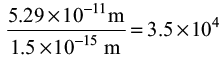5. Mass percent of Na in Na2CO3 is
(a) 21.52%
(b) 31.20%
(c) 38.20%
(d) 43.40%
Ans:
d
Solution: Mass percent of Na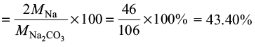6. Select the quantity of NO2 with highest mass:
(a) 100 amu
(b) 1.0 x 10–3 g
(c) 7.0 x 1022 molecules
(d) 8.0 x 10–1 mol
Ans: d
Solution: 100 amu = (100)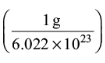= 1.66 x 10–22 g
Mass of 7.0 x 1022 molecules =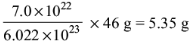Mass of 8.0 x 10–1 mol = 0.8 x 46 g = 36.8 g

Question 8:The volume of concentrated sulphuric acid (98 mass % H2SO4, density 1.84 g cm–3) required to prepare 5 dm3 of 0.5 mol dm–3 solution of sulphuric acid is

Question 9:Iron has a density of 7.86 g cm–3 and an atomic mass of 55.85 amu. The volume occupied by 1 mol of Fe is

Question 10:The combustion of 4.24 mg of an organic compound produces 8.45 mg of CO2 and 3.46 mg of water. The mass percentages of C and H in the compound, respectively, are

Question 11:The simplest formula of the compound containing 32.5% K, 0.839% H, 26.7% S and 39.9% O by mass is

Question 12:If in a reaction HNO3 is reduced to NO, the mass of HNO3 absorbing one mole of electrons would be

Question 13:The SI unit of magnetic flux density is

Question 14:Boron occurs in two varieties, namely, 10B (atomic mass: 10.01 amu) 11B (atomic mass: 11.01 amu). The atomic mass of the naturally occurring element is reported as 10.82 amu. The percent of 10B in this naturally occurring boron is

7. The volume of 0.25 M NaOH to be added to 250 mL of 0.15 M NaOH so that the resultant solution is 0.2 M would be
(a) 250 mL
(b) 350 mL
(c) 450 mL
(d) 550 mL
Ans: a
Solution: Let V be the volume of 0.25 M NaOH solution. Total amount of NaOH after mixing the two solutions is n = V (0.25 mol L–1) + (0.25 L) (0.15 mol L–1)
Total volume of the solution = V + 0.25 L
Molarity of the resultant solution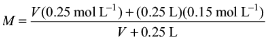Equating this to 0.2 M, we get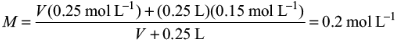Solving for V, we get V = 0.25 L = 250 mL

8. The mass of H2O2 that is completely oxidised by 30.2 g of KMnO4 (molar mass = 158 g mol–1) in acidic medium is
(a) 12 g
(b) 15 g
(c) 17 g
(d) 1 g
Ans:
c
Solution: The reaction is 2KMnO4 + 5H2O2 + 6H+ → 2Mn2+ + 5O2 + 8H2O + 2K+
2 x 151 g of KMnO4 reacts completely with 5 x 34 g of H2O2
30.2 g of KMnO4 reacts completely with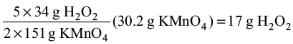Question 15:The sulphur in 1.0 g sample of steel is burned to sulphur dioxide and absorbed in 50.0 mL of 0.1 M sodium hydroxide solution. The excess sodium hydroxide requires 24.0 mL of 0.15 M hydrochloric acid solution for neutralization. The percentage of sulphur in the sample is

Question 16:A 0.50 g sample containing only anhydrous FeCl3 (molar mass: 162.5 g mol-1) and AlCl3 (molar mass: 133.5 g mol-1) yielded 1.435 g of AgCl (molar mass: 143.5 g mol-1). The mass of FeCl3 in the sample is

Question 17:The volume of water needs to be added to 10.0 mL of nitric acid (density: 1.40 g ml-1) containing 70 mass percent of acid to prepare 1.0 M solution would be

Offer running on EduRev: Apply code STAYHOME200 to get INR 200 off on our premium plan EduRev Infinity!

## Chemistry for JEE

223 videos|452 docs|334 tests

,

,

,

,

,

,

,

,

,

,

,

,

,

,

,

,

,

,

,

,

,

;